Governors' Area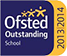# Mathematics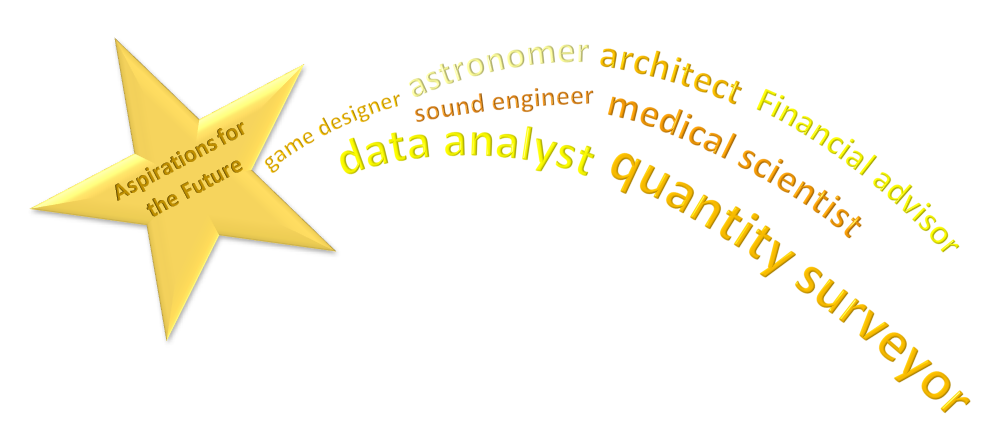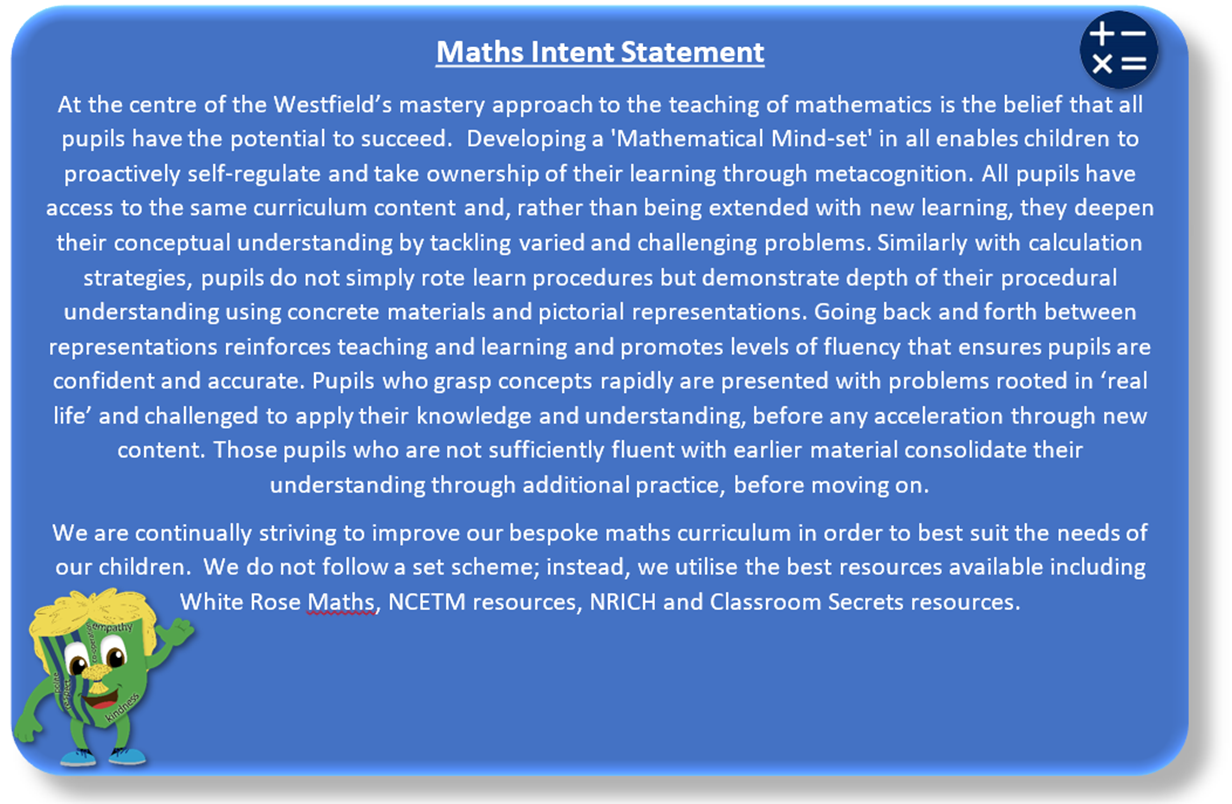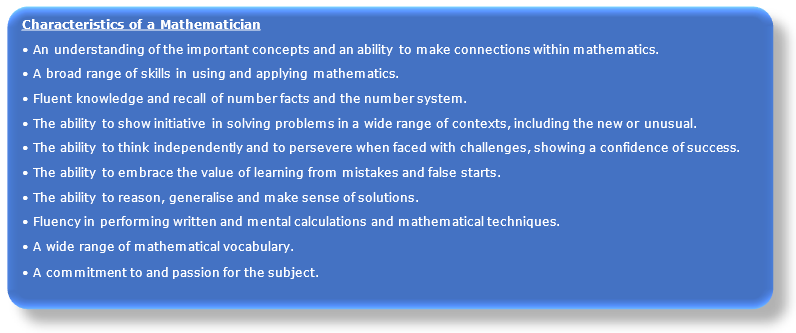Implementation

At Bourne Westfield, children are taught under these learning concepts:

• Know and use numbers

This concept involves understanding the number system and how they are used in a wide variety of mathematical ways.

This concept involves understanding both the concepts and processes of addition and subtraction.

• Multiply and divide

This concept involves understanding both the concepts and processes of multiplication and division.

• Use fractions

This concept involves understanding the concept of part and whole and ways of calculating using it.

• Understand the properties of shapes

This concept involves recognising the names and properties of geometric shapes and angles.

• Describe position, direction and movement

This concept involves recognising various types of mathematical movements.

• Use measures

This concept involves becoming familiar with a range of measures, devices used for measuring and calculations.

• Use statistics

This concept involves interpreting, manipulating and presenting data in various ways.

• Use algebra
• This concept involves recognising mathematical properties and relationships using symbolic representations

We encourage children to talk like mathematicians using the sentence stems below.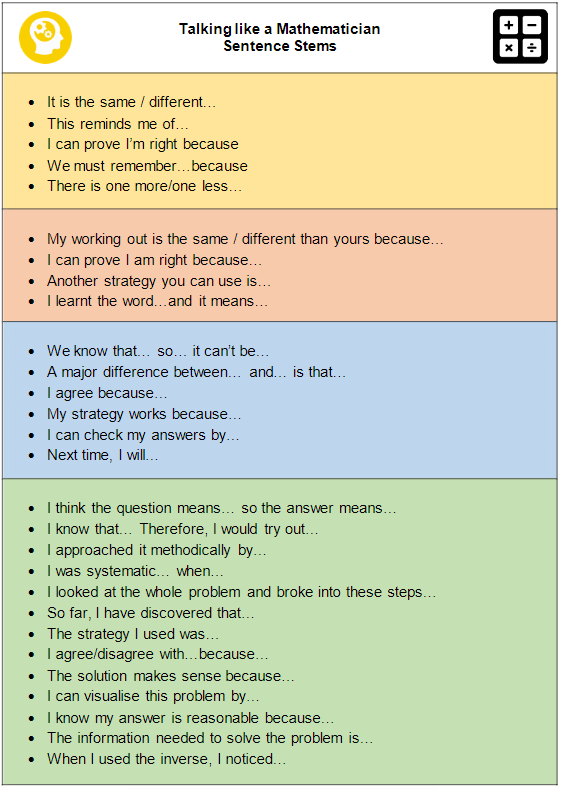Maths Progression - White Rose

Helpful Parent Guides and Resources are available. Please click to visit the White Rose site.